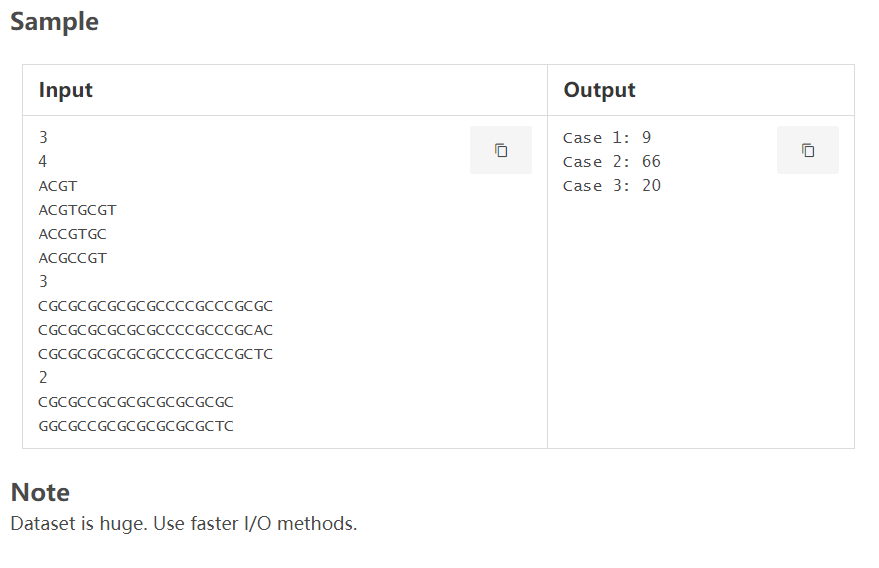# LightOJ DNA Prefix（Trie）

Given a set of n DNA samples, where each sample is a string containing characters from {A, C, G, T}, we are trying to find a subset of samples in the set, where the length of the longest common prefix multiplied by the number of samples in that subset is maximum.

To be specific, let the samples be:

1. ACGT
2. ACGTGCGT
3. ACCGTGC
4. ACGCCGT

If we take the subset {ACGT} then the result is 4 (4 * 1), if we take {ACGT, ACGTGCGT, ACGCCGT} then the result is 3 * 3 = 9 (since ACG is the common prefix), if we take {ACGT, ACGTGCGT, ACCGTGC, ACGCCGT} then the result is 2 * 4 = 8.

Now your task is to report the maximum result we can get from the samples.

Input

Input starts with an integer T (≤ 10), denoting the number of test cases.

Each case starts with a line containing an integer n (1 ≤ n ≤ 50000) denoting the number of DNA samples. Each of the next n lines contains a non empty string whose length is not greater than 50. And the strings contain characters from {A, C, G, T}.

Output

For each case, print the case number and the maximum result that can be obtained.有 t 组测试样例，每组测试给出 n 个字符串，求这 n 个字符串的（公共前缀*公共前缀的长度）的最大值

const int N=2e6+5e5+5;

int n,m;
int i,j,k;
char s;
int t[N];
int sum[N],tot=0;
map<char,int> mp;
int ans=0;

int idx(char ch){ return mp[ch]; }

void insert(char *s,int rt)
{
for(int i=0;s[i];i++){
int x=idx(s[i]);
if(!t[rt][x]) t[rt][x]=++tot;
rt=t[rt][x];
sum[rt]++;
if(sum[rt]) ans=max(ans,(i+1)*sum[rt]);
}
}

void clear()
{
for(int i=0;i<=tot;i++){
sum[i]=0;
for(int j=0;j<4;j++) t[i][j]=0;
}
ans=0,tot=0;
}

int main()
{
//IOS;
int num=0;
mp['G']=0,mp['A']=1,mp['C']=2,mp['T']=3;
rush(){
sd(n);
for(int i=1;i<=n;i++) ss(s),insert(s,0);
printf("Case %d: %d\n",++num,ans);
clear();
}
PAUSE;
return 0;
}

10-04206
07-162611-03522
06-12173
09-0649
08-04556
03-10450
08-23374
08-1350
04-19
09-28
06-13